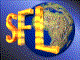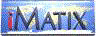| iMatix home page | << | < | > | >>SFLVersion 2.11

### tree_delete

```#include "sfltree.h"
void tree_delete (TREE **root, void *tree)
```

#### Synopsis

Deletes a node from a tree. Does not deallocate any memory.

#### Source Code - (sfltree.c)

```{
TREE
*youngest, *descendent;
TREE_COLOUR
colour;

if ((!tree)
||  (tree == TREE_NULL))
return;

if ((((TREE *) tree)-> left  == TREE_NULL)
||  (((TREE *) tree)-> right == TREE_NULL))
/* descendent has a TREE_NULL node as a child */
descendent = tree;
else
{
/* find tree successor with a TREE_NULL node as a child */
descendent = ((TREE *) tree)-> right;
while (descendent-> left != TREE_NULL)
descendent = descendent-> left;
}

/* youngest is descendent's only child, if there is one, else TREE_NULL */
if (descendent-> left != TREE_NULL)
youngest = descendent-> left;
else
youngest = descendent-> right;

/* remove descendent from the parent chain */
if (youngest != TREE_NULL)
youngest-> parent = descendent-> parent;
if (descendent-> parent)
if (descendent == descendent-> parent-> left)
descendent-> parent-> left  = youngest;
else
descendent-> parent-> right = youngest;
else
*root = youngest;

colour = descendent-> colour;

if (descendent != (TREE *) tree)
{
/* Conceptually what we are doing here is moving the data from       */
/* descendent to tree.  In fact we do this by linking descendent     */
/* into the structure in the place of tree.                          */
descendent-> left   = ((TREE *) tree)-> left;
descendent-> right  = ((TREE *) tree)-> right;
descendent-> parent = ((TREE *) tree)-> parent;
descendent-> colour = ((TREE *) tree)-> colour;

if (descendent-> parent)
{
if (tree == descendent-> parent-> left)
descendent-> parent-> left  = descendent;
else
descendent-> parent-> right = descendent;
}
else
*root = descendent;

if (descendent-> left != TREE_NULL)
descendent-> left-> parent = descendent;

if (descendent-> right != TREE_NULL)
descendent-> right-> parent = descendent;
}

if ((youngest != TREE_NULL)
&&  (colour   == BLACK))
delete_fixup (root, youngest);
}
```

 | << | < | > | >>Copyright © 1996-2000 iMatix Corporation